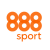## FRACTIONAL ODDS EXPLAINED

Whenever you see two numbers separated by a slash, i.e. 4/1, this is a fractional betting odd. The number on the right is the unit stake you are betting in and the number on the left is the potential return per unit stake.

In the case of a 4/1 bet, your bet is £1 and if it is successful you will win £4. The total return on this bet would therefore be £5 (initial £1 stake plus £4 winnings).The probability of a 4/1 chance winning is 20% - based on his calculation 1 / (4 + 1) = 0.20

## DECIMAL ODDS EXPLAINED

Decimals are an alternative to seeing betting odds in the fractional format. Which option to view odds in is a personal preference for customers. Here is the calculation for decimal odds:

`Winnings = (odds * stake) - stake`

Decimal odds are written as 9.0 (which is the equivalent of 8/1 in fractional odds). When you see decimal odds displayed, remember that these include your unit stake of one.

If you bet £1 at 9.0 and your bet wins, you will get back £9 (initial £1 stake plus £8 winnings). The probability of a 9.0 chance winning is 10% - based on this calculation 1 / (9 + 1) = 0.10